Relativity and Cosmology Blog# Relativity and Cosmology

This is a Blog on relativity and cosmology for engineers and the like. My website "Relativity-4-Engineers" has more in-depth stuff.

Comments/questions of a general nature should preferably be posted to the FAQ section of this Blog (http://cr4.globalspec.com/blogentry/316/Relativity-Cosmology-FAQ).

A complete index to the Relativity and Cosmology Blog can be viewed here: http://cr4.globalspec.com/blog/browse/22/Relativity-and-Cosmology"

Regards, Jorrie

 Previous in Blog: Cosmology Equations Part 3 Next in Blog: Cosmology Equations Part 5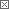# Cosmology Equations Part 4

Posted November 05, 2006 11:00 AM by Jorrie
Pathfinder Tags: cosmology Friedmann

This mini-article deals with the much celebrated Friedmann equation of cosmological expansion. In Part 3 of this mini-series, we had a brief look at the cosmological energy density parameter as a function of the expansion factor (a):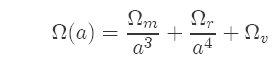This naturally leads on to the Friedmann equation for the expansion rate of the universe over time: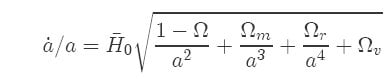Formidable as it looks, it is actually quite simple to understand, if taken step by step: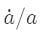is the rate of change of the expansion factor per unit expansion factor. Recall that the expansion factor a is the ratio between how much expansion has happened at any time and the amount of expansion today. The present value of a = 1 and at the Big Bang it was zero.is somewhat like expressing your car's speed as a fraction of the distance covered. If you drive at a a constant speed, the fraction will shrink as distance covered gets larger. The reason for this unusual ratio will soon become apparent.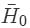(pronounced H-naught-bar) is the Hubble constant, the present expansion rate of the universe. Note that a and all the Ωs are dimensionless, so a-dot and H0-bar must have the same units, which is inverse time (time-1). The bar is just to indicate that it is a normalized expansion rate and not the conventional H0 expressed in conventional units (Km s-1 Mpc-1), where Mpc is Mega-parsec.

The square root is a function of the expansion factor and shrinks as a grows larger. Ω is the present total energy density parameter, where Ω = Ωm+ Ωr + Ωv ≈ 1, meaning that the total energy density is very close to the critical density, giving a flat universe.

The other values inside the square root have been discussed in Part 3 and will just be briefly noted: Ωm, Ωr, Ωv are mass energy density, radiation energy density and vacuum energy density respectively.

So what does the Friedmann equation really tell us? (We will reproduce it here, otherwise it tends to scroll off the top...)The graph below plots the value of the square root as a function of a and the constants: Ωr = 0.001, Ωm= 0.2999, Ωv = 0.7 (so that Ω = 1). For the individual components, it was calculated as if only that component is present, e.g., for matter density, the purple curve, SQRT(Ωm/a3) was plotted.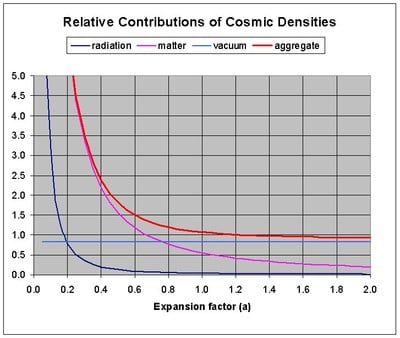When a << 1, Ωr/a4 (radiation energy density, the dark blue curve) dominated the expansion. A fraction with a very small value to the power 4 below the line can result in a very large value. Due to the scale requirements, it's not very visible on the graph, but just look at the relative slopes and you can easily see that the radiation part will dominate all else for very small a.

As a became larger then about 0.05 (crossover also not visible on the scale of the graph), matter density started to dominate, because Ωm>> Ωr. According to our best knowledge, this matter domination phase lasted for more than half the history of the universe.

As a grows larger than about 0.8, and if vacuum energy density is around Ωv = 0.7, it starts to dominate the value inside the square root. It is easy to see that the other terms under the root get 'diluted' by a > 1. Actually, the square root part will approach the constant value: sqrt(Ωv) asymptotically as a grows very large.

So much for the right hand side of the Friedmann equation. What does this tell us about the left hand side?

On the left hand side, a, however large, still features below the line. The only way that the left side can also achieve constancy for large a, is for the expansion rate (a-dot) to change in the same sense and magnitude (increasing or decreasing) than what a does.

This explains how the universe could gradually go over from an initially decreasing expansion rate to an increasing expansion rate, provided that there is vacuum energy (a.k.a. dark energy) with positive density.

Does all this tend to bend the mind a bit? Do not feel alone! The cosmology part of the website Relativity 4 Engineers attempts to make it more digestible for engineers. Or you can read Peebles or Peacock (post-grad physical cosmology books)...

Next - how to plot a cosmological expansion curve, using the Friedmann equation...

Interested in this topic? By joining CR4 you can "subscribe" to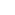#1

###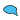Re: Cosmology Equations Part 4

11/08/2006 9:53 AM

Great post as usual. I have several questions:

1. Where do the values of Ωr, Ωm, and Ωv come from? Are they determined through
observation or theory?

2. If a/a was less than one during the early phases of the Universe, does that imply the universe at the time was not flat but curved in some manner? How much?

3. Since the Hubble Constant is in units of inverse time, does that imply that the change in expansion rate is linear? What does that imply about how Ωr, Ωm, and Ωv are changing (exponentially?) (I'm not sure if this question makes any sense)#2

###Re: Cosmology Equations Part 4

11/08/2006 12:51 PM

Hi Roger, some rather tentative answers to difficult questions!

"1. Where do the values of Ωr, Ωm, and Ωv come from? Are they determined through
observation or theory
?"

Ωr and Ωm are measured more or less directly, by measuring (estimating) the matter and radiation from a given volume of large scale space. Ωv is measured indirectly by means of various collaborating observations and the result is in fact somewhat expansion model dependant.

2. "If a/a was less than one during the early phases of the Universe, does that imply the universe at the time was not flat but curved in some manner? How much?"

I'm afraid it's nor clear what you mean, because a/a =1, a-dot/a was very, very large in the early phases and a was very small - please clarify.

3. "Since the Hubble Constant is in units of inverse time, does that imply that the change in expansion rate is linear? What does that imply about how Ωr, Ωm, and Ωv are changing (exponentially?) (I'm not sure if this question makes any sense)"

I don't think the change in expansion rate (a-dot) is thought to be linear, because a itself apparently changed from negative in the 'middle ages' of the universe to positive at present, but I'm not sure - I'll look into it a bit and come back to you. Jorrie

__________________
"Perplexity is the beginning of knowledge." -- Kahlil Gibran#3

###Re: Cosmology Equations Part 4

11/08/2006 1:15 PM

Thanks for the answers, I'll try to clarify my questions.

1. I think you've answered this one for me.

2. I think I misunderstood a few things and that led to a bad question, let me rephrase this question as "Has Ω (total) always been about 1 or was it a different value in the past? If it was a different value in the past, does this mean the universe wasn't always flat?

3. If the Hubble constant is constant, than a-dot/a has to be constant as well if Ω(total) has always been 1, otherwise Ω(total) has to have changed or the Hubble constant isn't a constant? Do we know which it is?

Please let me know, I find this subject extremely interesting and I'd really like to understand it correctly.#4

###Re: Cosmology Equations Part 4

11/08/2006 10:58 PM

Hi Roger,

2. If Ω ≈ 1 today, as measured, it must have been much closer to 1 in the past, something like deviating less 1 in 1060 from unity at the end of inflation! In my book R4E there is a subsection on it on page 187, s/s 14.5; or browse for the pdf on my site under Cosmology, Friedmann equation.

3. No, a-dot/a has not always been constant - it is only in the far future, with a >> 1, that this may happen. Just after inflation a-dot/a was astronomically large, because a was very small inside that square root.